MORE IN Dynamics of Machinery
SPPU Mechanical Engineering (Semester 7)
Dynamics of Machinery
June 2015
Total marks: --
Total time: --
INSTRUCTIONS
(1) Assume appropriate data and state your reasons
(2) Marks are given to the right of every question
(3) Draw neat diagrams wherever necessary

Answer any one question from Q1 and Q2
1 (a) Explain clearly the terms static and dynamic balancing.
4 M
1 (b) A shaft carries four masses A,B,C and D which are placed in parallel planes perpendicular to the longitudinal axis. The unbalanced masses at planes B and C are 3.6kg and 2.5kg respectively and both are assumed to be concentrated at a radius of 25mm while the masses in planes A and D are both at radius of 40mm. The angle between the planes B and C is 100 o and that between B and A is 190°, both angles being measured in counter clockwise direction from the plane B. the planes containing A and B are 250mm apart and those containing B and C are 500mm. If the shaft is to be completely balanced, determine:
i) Masses at the planes A and D
ii) The distance between the planes C and D
iii) The angular position of mass D
12 M

2 (a) What do you mean by balancing machine? Explain any one type of static balancing machine.
6 M
2 (b) A three cylinder radial engine has axes at 120 o to one another and their connecting rods are coupled to a single common crank. The stroke length is 100mm and length of each connecting rod is 150mm. If the mass of reciprocating parts per cylinder is 1 kg, determine the primary and secondary force of the engine running at 2400 RPM.
10 M

Answer any one question from Q3 and Q4
3 (a) With neat sketches explain underdamped, over damped and critically damped systems.
3 M
3 (b) A vibrating system is defined by the following parameters:
m = 3 kg, k = 100 N/m, c = 3 N-s/m
Determine:
i) The damping factor
ii) The natural frequency of damped vibration
iii) Logarithmic decrement
iv) The ratio of two consecutive amplitudes
v) The number of cycles after which the original amplitude is reduced to 20%.
5 M
3 (c) For the system shown in Fig. No. 01, find the equation of motion and also determine its natural frequency.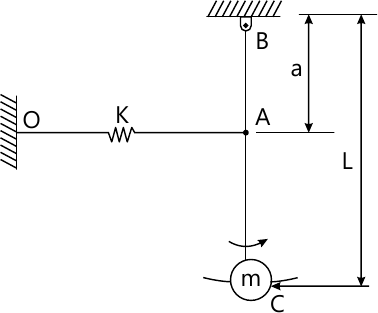8 M

4 (a) What is logarithmic decrement? Derive an expression for the same.
6 M
4 (b) A homogeneous solid cylinder of mass 'm' is linked by a spring of constant k' N/m. If it rolls without slipping, show that frequency of oscillation is [ sqrt{ dfrac {2k}{3m} } rad/s ]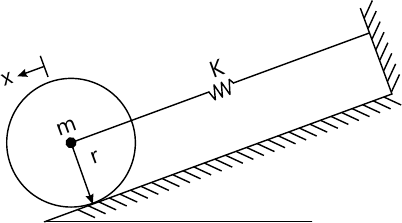5 M
4 (c) An under damped shock absorber is to be designed for an automobile. It is required that initial amplitude to be reduced to 1/16th in one cycle. The mass of the automobile is 200kg and damped period of vibration is 1 sec. Find necessary stiffness and damping constants of shock absorbers.
5 M

Answer any one question from Q5 and Q6
5 (a) The damped frequency of a system as obtained from a free vibration test is 10.5Hz. During the forced vibration test with constant excitation force on the same system, the peak frequency of vibration is found to be at 9.5Hz. Find the damping factor of the system and its natural frequency.
4 M
5 (b) Neatly plot the frequency response curves and draw any four conclusions from the same.
6 M
5 (c) A system having rotating unbalance has total mass of 25kg. The unbalanced mass of 1kg rotates with a radius 0.04m. It has been observed that at a speed of 1000RPM, the system and eccentric mass have a phase difference of 90° and the corresponding amplitude is 0.015m. Find out:
i) Natural frequency of the system
ii) Damping factor
iii) Amplitude at 1500 RPM
iv) Phase angle at 1500 RPM
8 M

6 (a) A vehicle has a mass 490kg and the total spring constant of its suspension system is 58,800 N/m. The profile of the road may be approximated to a sine wave of amplitude 40mm and wavelength 4m, as shown in fig no 03. Determine:
i) Critical speed of the vehicle
ii) The amplitude of the steady state motion of the mass when the vehicle is driven at critical speed and ξ= 0.5.
iii) The amplitude of steady state motion of mass when the vehicle is driven at 57 km/hr and damping factor = 0.5.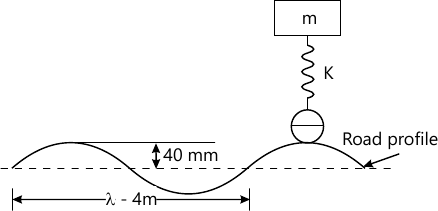9 M
6 (b) Explain the following terms.
i) Vibration isolation
ii) Force transmissibility
iii) Motion transmissibility
9 M

Answer any one question from Q7 and Q8
7 (a) Explain the concept of torsionally equivalent shaft.
6 M
7 (b) Derive the differential equations of motion for the system shown in figure no.04. It is given that m1 = 20Kg, m2 = 35Kg and k = 3000N/m. Determine
i) The natural frequencies
ii) Amplitude ratio for the two mode
iii) Principal mode shape.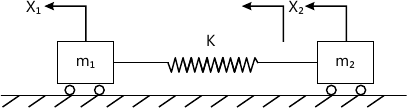12 M

8 (a) What do you mean by whirling of shaft? Derive the expression for deflection vertical shaft with a single rotor without damping.
6 M
8 (b) Two equal masses of weight 400N each and radius of gyration 40cm are keyed to the opposite ends of shaft 60cm long. The shaft is 7.5cm diameter for the first 25cm of its length, 12.5cm diameter for the next 10cm and 8.5cm diameter for the remaining of its length as shown in figure no. 05. Find the natural frequency of the torsionally vibration of the system and position of node. Assume 0.84×1011 N/m2.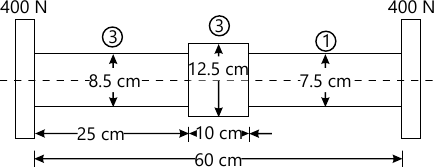12 M

Answer any one question from Q9 and Q10
9 (a) Explain human hearing mechanism with sketch.
4 M
9 (b) Explain the term sound reflection, Absorption, and Transmission.
6 M
9 (c) What do you understand by sound enclosure? Describe two types of sound enclosure.
6 M

10 (a) Explain the working of microphone.
4 M
10 (b) Define sound field. Explain the various types of sound field in the vicinity of some sources.
6 M
10 (c) A mechanism working in machine shop is operating having there sound pressure levels as 95dB, 90dB, 92dB, 88dB and 83dB respectively. Determine total sound level when
i) All mechanism are ON
ii) Machine 2 and 3 are turned OFF.
6 M

Answer any one question from Q11 and Q12
11 (a) Explain general vibration measurement process with neat sketch.
8 M
11 (b) What do you understand by the time domain and frequency domain? How are they useful in predicting vibration failures?
8 M

Write short notes on the following:
12 (a) Piezo electric accelerometer.
5 M
12 (b) Vibration exciter.
6 M
12 (c) FFT analyzer.
5 M

More question papers from Dynamics of Machinery